Скачать презентацию COMP 3221 Microprocessors and Embedded Systems Lectures 20

d1787db9e950685381cc85da575f60a0.ppt

• Количество слайдов: 28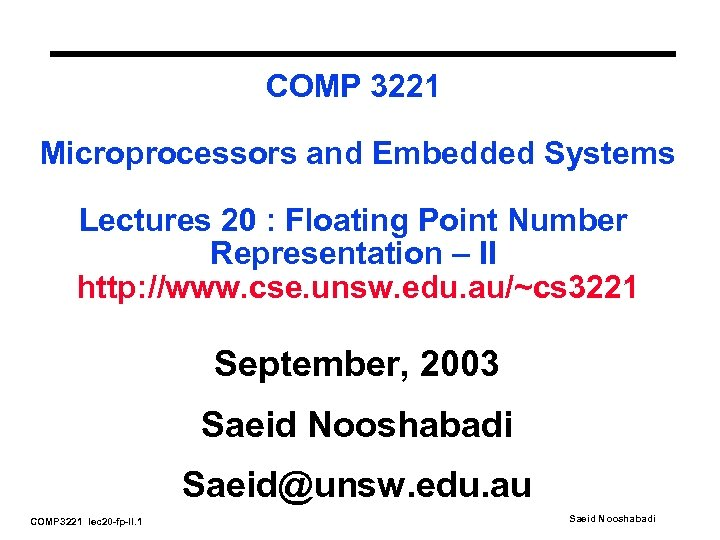COMP 3221 Microprocessors and Embedded Systems Lectures 20 : Floating Point Number Representation – II http: //www. cse. unsw. edu. au/~cs 3221 September, 2003 Saeid Nooshabadi [email protected] edu. au COMP 3221 lec 20 -fp-II. 1 Saeid Nooshabadi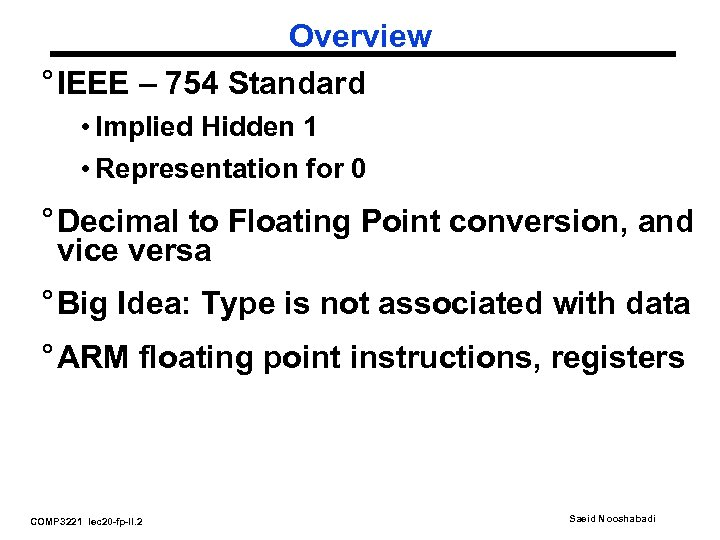Overview ° IEEE – 754 Standard • Implied Hidden 1 • Representation for 0 ° Decimal to Floating Point conversion, and vice versa ° Big Idea: Type is not associated with data ° ARM floating point instructions, registers COMP 3221 lec 20 -fp-II. 2 Saeid Nooshabadi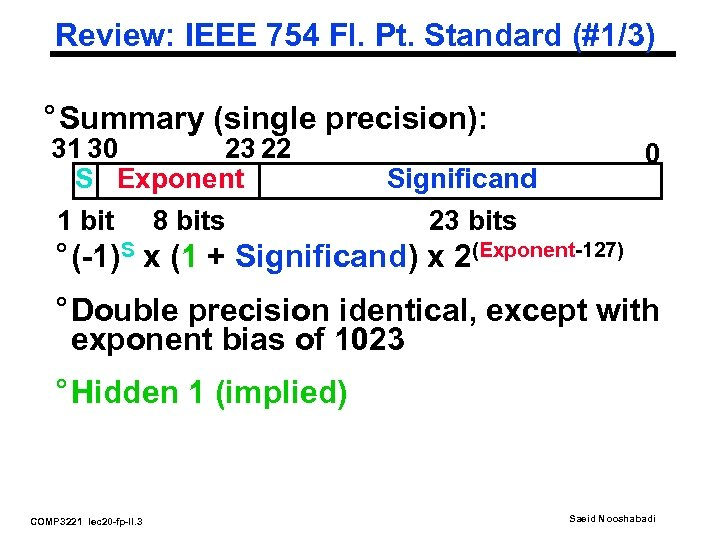Review: IEEE 754 Fl. Pt. Standard (#1/3) ° Summary (single precision): 31 30 23 22 S Exponent 1 bit 8 bits 0 Significand 23 bits ° (-1)S x (1 + Significand) x 2(Exponent-127) ° Double precision identical, except with exponent bias of 1023 ° Hidden 1 (implied) COMP 3221 lec 20 -fp-II. 3 Saeid Nooshabadi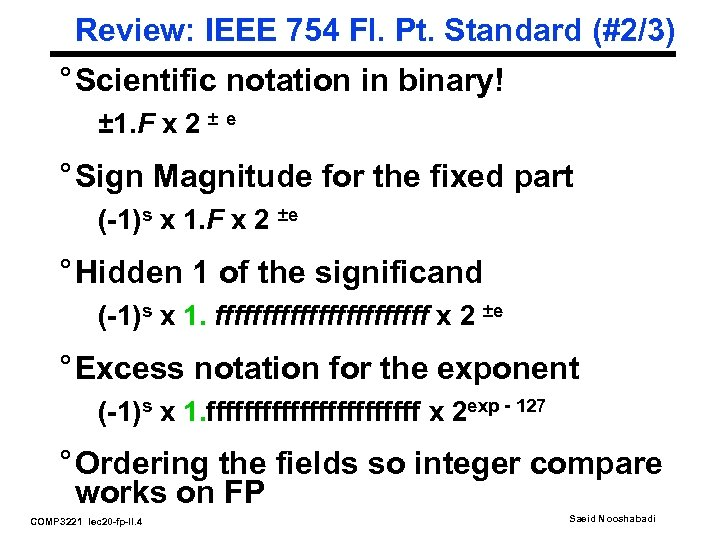Review: IEEE 754 Fl. Pt. Standard (#2/3) ° Scientific notation in binary! ± 1. F x 2 ± e ° Sign Magnitude for the fixed part (-1)s x 1. F x 2 ±e ° Hidden 1 of the significand (-1)s x 1. ffffffffffff x 2 ±e ° Excess notation for the exponent (-1)s x 1. ffffffffffff x 2 exp - 127 ° Ordering the fields so integer compare works on FP COMP 3221 lec 20 -fp-II. 4 Saeid Nooshabadi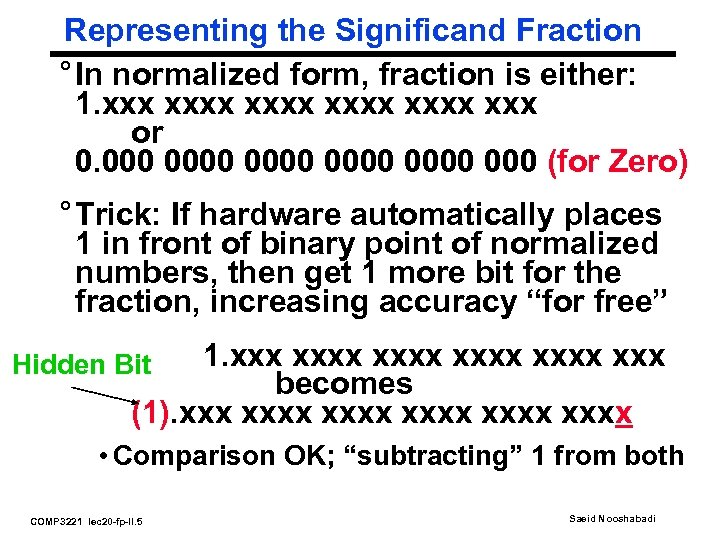Representing the Significand Fraction ° In normalized form, fraction is either: 1. xxxx xxx or 0. 0000 000 (for Zero) ° Trick: If hardware automatically places 1 in front of binary point of normalized numbers, then get 1 more bit for the fraction, increasing accuracy “for free” 1. xxxx xxx becomes (1). xxxx xxxx Hidden Bit • Comparison OK; “subtracting” 1 from both COMP 3221 lec 20 -fp-II. 5 Saeid Nooshabadi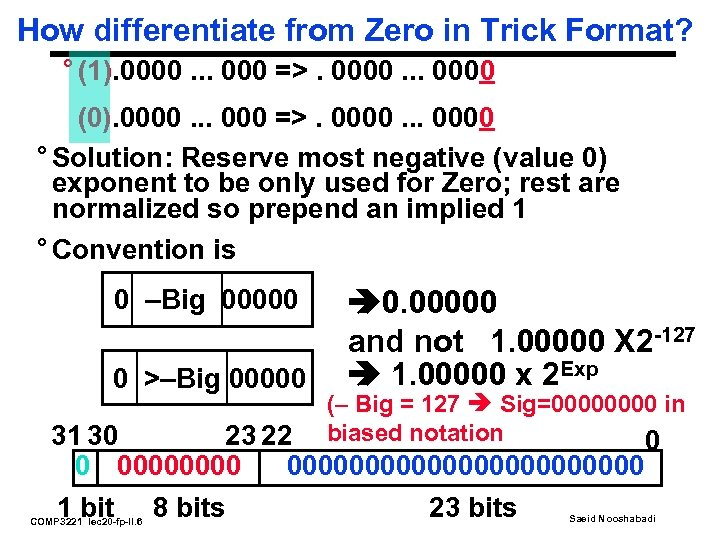How differentiate from Zero in Trick Format? ° (1). 0000. . . 000 =>. 0000. . . 0000 (0). 0000. . . 000 =>. 0000. . . 0000 ° Solution: Reserve most negative (value 0) exponent to be only used for Zero; rest are normalized so prepend an implied 1 ° Convention is 0 –Big 00000 0 >–Big 00000 0. 00000 and not 1. 00000 X 2 -127 1. 00000 x 2 Exp (– Big = 127 Sig=0000 in biased notation 0 31 30 23 22 0 000000000000000 1 bit 8 bits 23 bits COMP 3221 lec 20 -fp-II. 6 Saeid Nooshabadi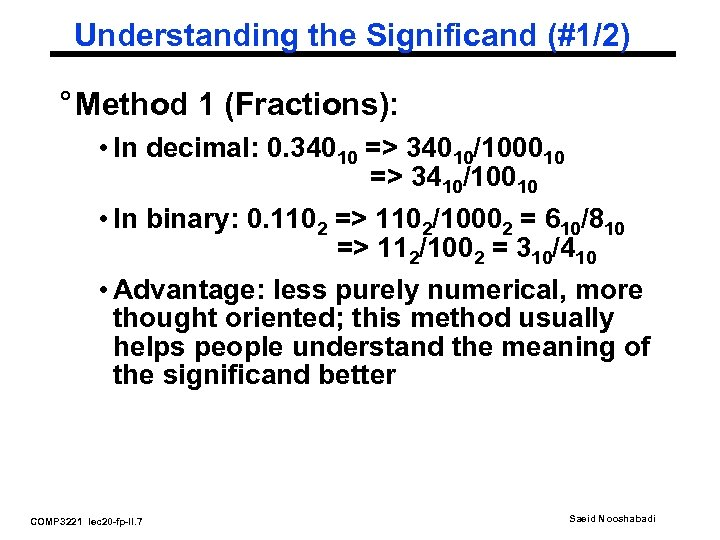Understanding the Significand (#1/2) ° Method 1 (Fractions): • In decimal: 0. 34010 => 34010/100010 => 3410/10010 • In binary: 0. 1102 => 1102/10002 = 610/810 => 112/1002 = 310/410 • Advantage: less purely numerical, more thought oriented; this method usually helps people understand the meaning of the significand better COMP 3221 lec 20 -fp-II. 7 Saeid Nooshabadi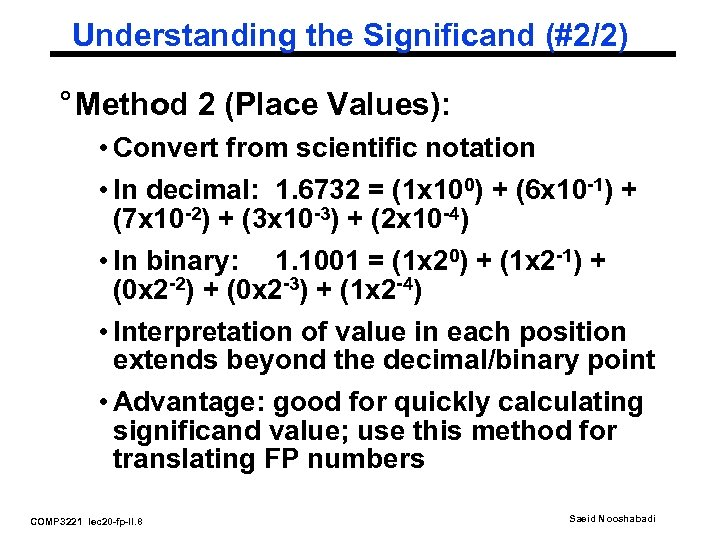Understanding the Significand (#2/2) ° Method 2 (Place Values): • Convert from scientific notation • In decimal: 1. 6732 = (1 x 100) + (6 x 10 -1) + (7 x 10 -2) + (3 x 10 -3) + (2 x 10 -4) • In binary: 1. 1001 = (1 x 20) + (1 x 2 -1) + (0 x 2 -2) + (0 x 2 -3) + (1 x 2 -4) • Interpretation of value in each position extends beyond the decimal/binary point • Advantage: good for quickly calculating significand value; use this method for translating FP numbers COMP 3221 lec 20 -fp-II. 8 Saeid Nooshabadi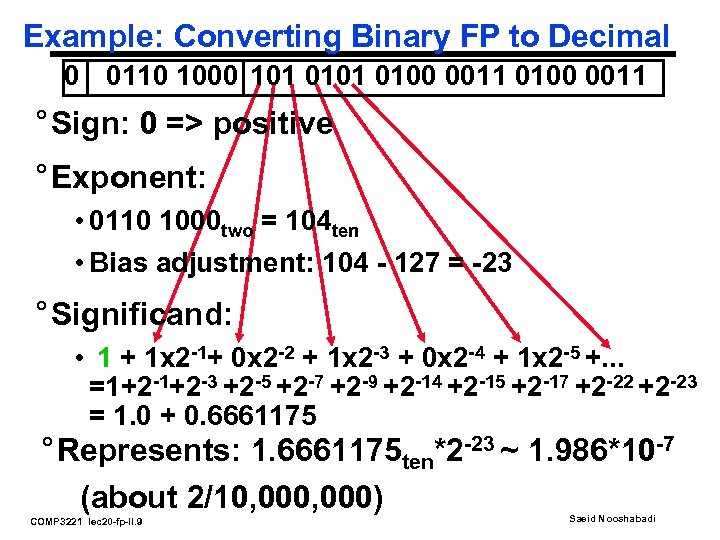Example: Converting Binary FP to Decimal 0 0110 1000 101 0100 0011 ° Sign: 0 => positive ° Exponent: • 0110 1000 two = 104 ten • Bias adjustment: 104 - 127 = -23 ° Significand: • 1 + 1 x 2 -1+ 0 x 2 -2 + 1 x 2 -3 + 0 x 2 -4 + 1 x 2 -5 +. . . =1+2 -3 +2 -5 +2 -7 +2 -9 +2 -14 +2 -15 +2 -17 +2 -22 +2 -23 = 1. 0 + 0. 6661175 ° Represents: 1. 6661175 ten*2 -23 ~ 1. 986*10 -7 (about 2/10, 000) COMP 3221 lec 20 -fp-II. 9 Saeid Nooshabadi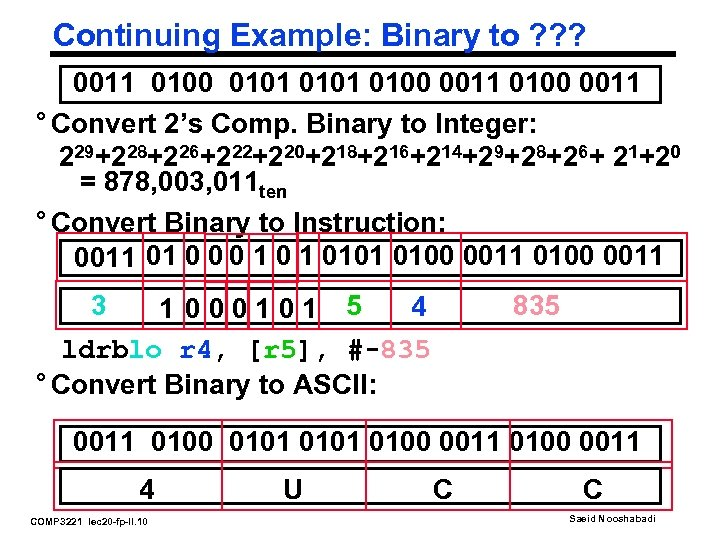Continuing Example: Binary to ? ? ? 0011 0100 0101 0100 0011 ° Convert 2’s Comp. Binary to Integer: 229+228+226+222+220+218+216+214+29+28+26+ 21+20 = 878, 003, 011 ten ° Convert Binary to Instruction: 0011 01 0 0 0 1 0101 0100 0011 3 835 4 1 000101 5 ldrblo r 4, [r 5], #-835 ° Convert Binary to ASCII: 0011 0100 0101 0100 0011 4 COMP 3221 lec 20 -fp-II. 10 U C C Saeid Nooshabadi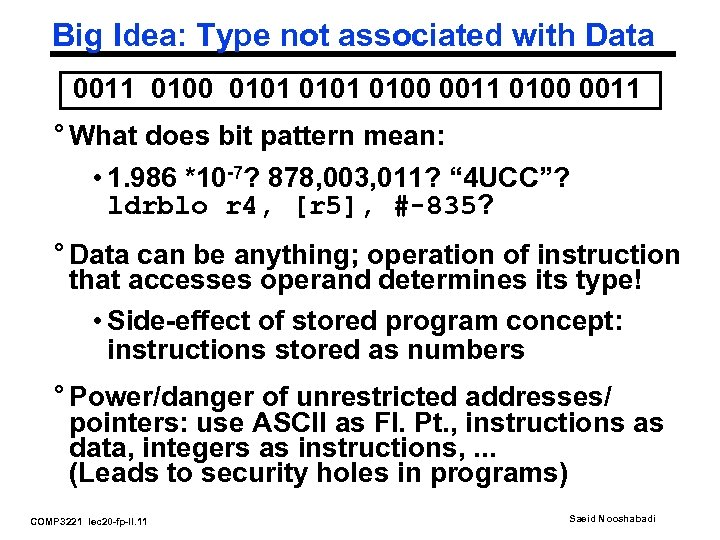Big Idea: Type not associated with Data 0011 0100 0101 0100 0011 ° What does bit pattern mean: • 1. 986 *10 -7? 878, 003, 011? “ 4 UCC”? ldrblo r 4, [r 5], #-835? ° Data can be anything; operation of instruction that accesses operand determines its type! • Side-effect of stored program concept: instructions stored as numbers ° Power/danger of unrestricted addresses/ pointers: use ASCII as Fl. Pt. , instructions as data, integers as instructions, . . . (Leads to security holes in programs) COMP 3221 lec 20 -fp-II. 11 Saeid Nooshabadi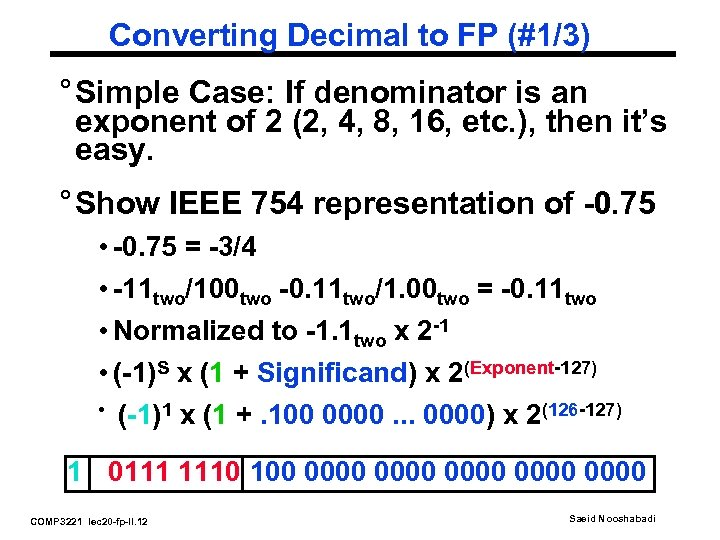Converting Decimal to FP (#1/3) ° Simple Case: If denominator is an exponent of 2 (2, 4, 8, 16, etc. ), then it’s easy. ° Show IEEE 754 representation of -0. 75 • -0. 75 = -3/4 • -11 two/100 two -0. 11 two/1. 00 two = -0. 11 two • Normalized to -1. 1 two x 2 -1 • (-1)S x (1 + Significand) x 2(Exponent-127) • (-1)1 x (1 +. 100 0000. . . 0000) x 2(126 -127) 1 0111 1110 100 0000 0000 COMP 3221 lec 20 -fp-II. 12 Saeid Nooshabadi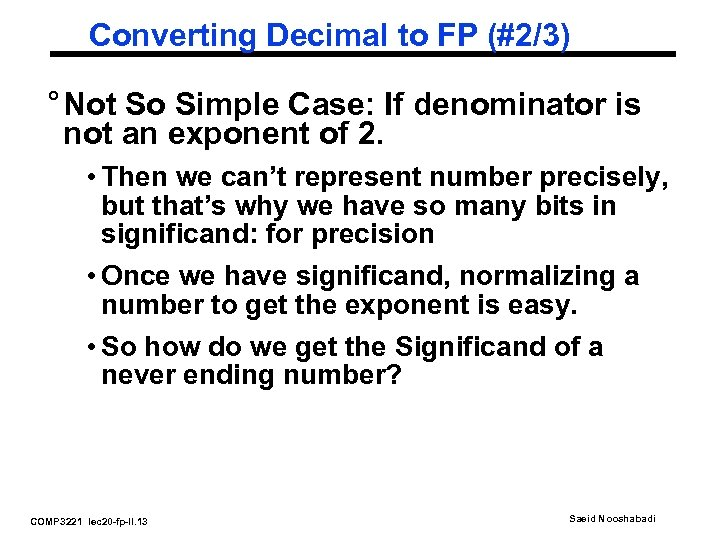Converting Decimal to FP (#2/3) ° Not So Simple Case: If denominator is not an exponent of 2. • Then we can’t represent number precisely, but that’s why we have so many bits in significand: for precision • Once we have significand, normalizing a number to get the exponent is easy. • So how do we get the Significand of a never ending number? COMP 3221 lec 20 -fp-II. 13 Saeid Nooshabadi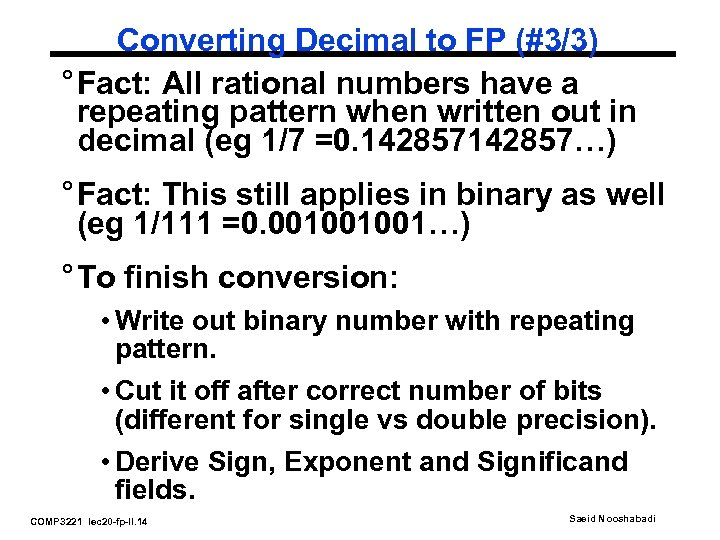Converting Decimal to FP (#3/3) ° Fact: All rational numbers have a repeating pattern when written out in decimal (eg 1/7 =0. 142857…) ° Fact: This still applies in binary as well (eg 1/111 =0. 001001001…) ° To finish conversion: • Write out binary number with repeating pattern. • Cut it off after correct number of bits (different for single vs double precision). • Derive Sign, Exponent and Significand fields. COMP 3221 lec 20 -fp-II. 14 Saeid Nooshabadi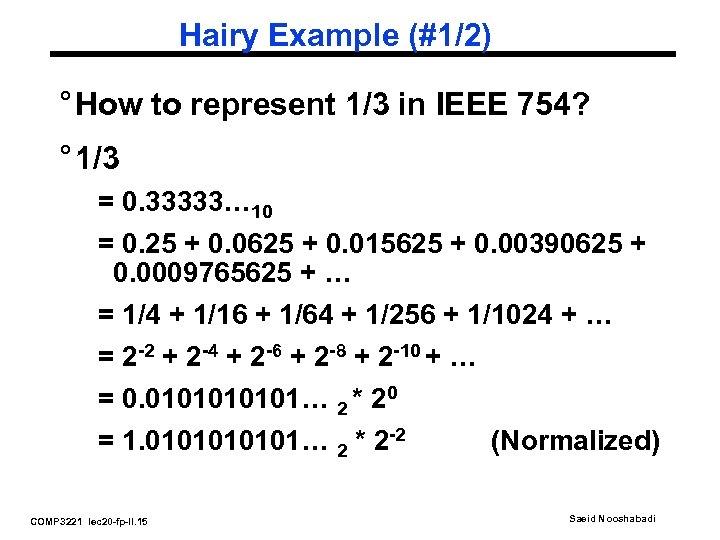Hairy Example (#1/2) ° How to represent 1/3 in IEEE 754? ° 1/3 = 0. 33333… 10 = 0. 25 + 0. 0625 + 0. 015625 + 0. 00390625 + 0. 0009765625 + … = 1/4 + 1/16 + 1/64 + 1/256 + 1/1024 + … = 2 -2 + 2 -4 + 2 -6 + 2 -8 + 2 -10 + … = 0. 010101… 2 * 20 = 1. 010101… 2 * 2 -2 (Normalized) COMP 3221 lec 20 -fp-II. 15 Saeid Nooshabadi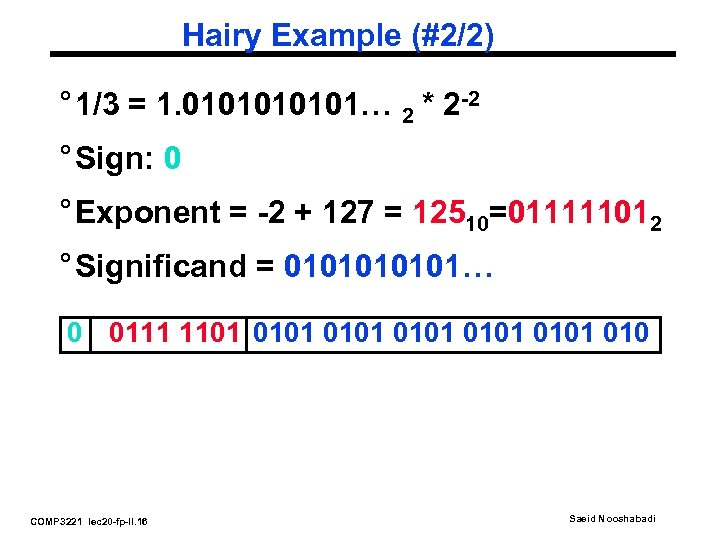Hairy Example (#2/2) ° 1/3 = 1. 010101… 2 * 2 -2 ° Sign: 0 ° Exponent = -2 + 127 = 12510=011111012 ° Significand = 010101… 0 0111 1101 0101 010 COMP 3221 lec 20 -fp-II. 16 Saeid NooshabadiWhat’s this stuff good for? Mow Lawn? ° Robot lawn mower: “Robomow RL-800” ° Surround lawn, trees with perimeter wire ° Sense tall grass to spin blades faster: up to 5800 RPM ° Slow if senses object, stop if bumps ° US\$700 Friendly Robotics of Even Yehuda, Israel, http: //www. robotic-lawnmower. com COMP 3221 lec 20 -fp-II. 17 Saeid Nooshabadi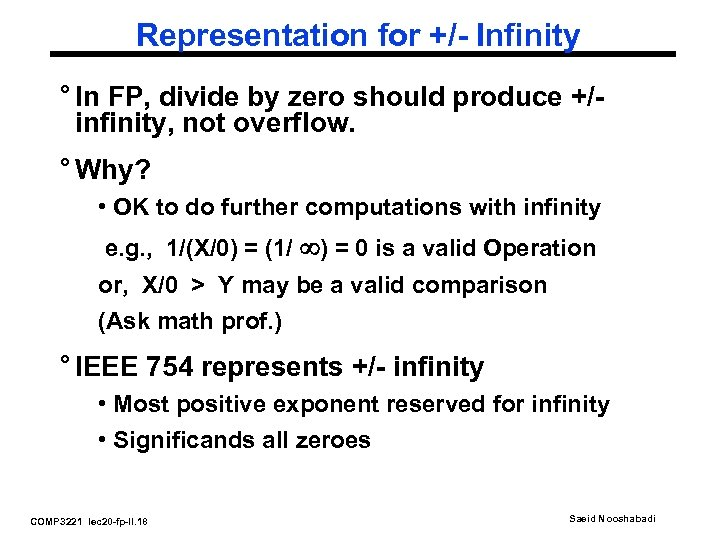Representation for +/- Infinity ° In FP, divide by zero should produce +/infinity, not overflow. ° Why? • OK to do further computations with infinity e. g. , 1/(X/0) = (1/ ) = 0 is a valid Operation or, X/0 > Y may be a valid comparison (Ask math prof. ) ° IEEE 754 represents +/- infinity • Most positive exponent reserved for infinity • Significands all zeroes COMP 3221 lec 20 -fp-II. 18 Saeid Nooshabadi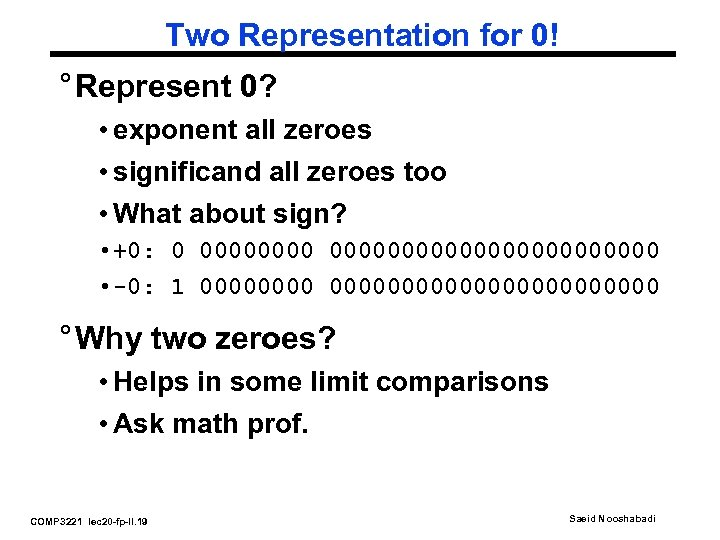Two Representation for 0! ° Represent 0? • exponent all zeroes • significand all zeroes too • What about sign? • +0: 0 000000000000000 • -0: 1 0000000000000000 ° Why two zeroes? • Helps in some limit comparisons • Ask math prof. COMP 3221 lec 20 -fp-II. 19 Saeid Nooshabadi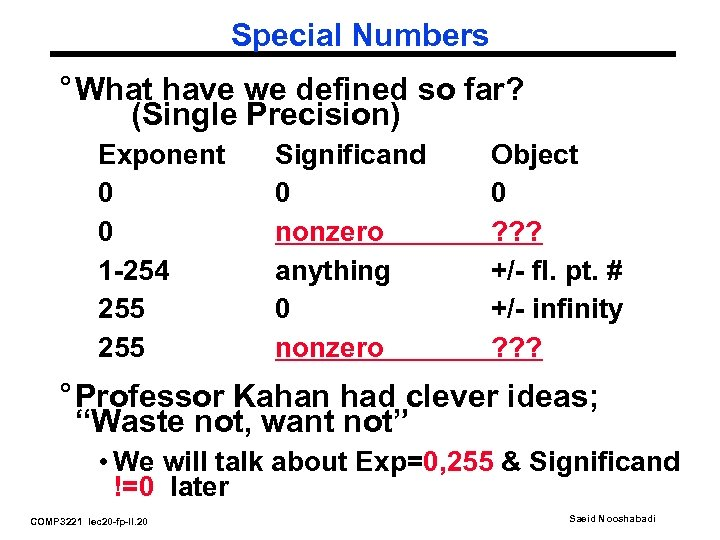Special Numbers ° What have we defined so far? (Single Precision) Exponent 0 0 1 -254 255 Significand 0 nonzero anything 0 nonzero Object 0 ? ? ? +/- fl. pt. # +/- infinity ? ? ? ° Professor Kahan had clever ideas; “Waste not, want not” • We will talk about Exp=0, 255 & Significand !=0 later COMP 3221 lec 20 -fp-II. 20 Saeid Nooshabadi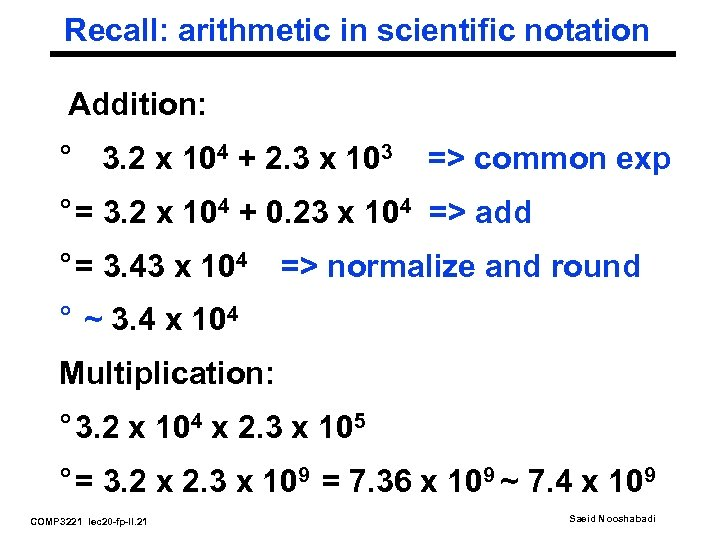Recall: arithmetic in scientific notation Addition: ° 3. 2 x 104 + 2. 3 x 103 => common exp ° = 3. 2 x 104 + 0. 23 x 104 => add ° = 3. 43 x 104 => normalize and round ° ~ 3. 4 x 104 Multiplication: ° 3. 2 x 104 x 2. 3 x 105 ° = 3. 2 x 2. 3 x 109 = 7. 36 x 109 ~ 7. 4 x 109 COMP 3221 lec 20 -fp-II. 21 Saeid Nooshabadi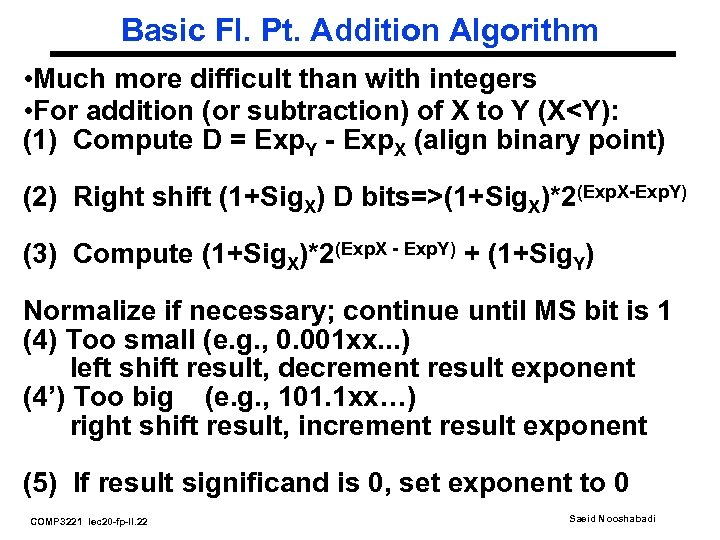Basic Fl. Pt. Addition Algorithm • Much more difficult than with integers • For addition (or subtraction) of X to Y (X(1+Sig. X)*2(Exp. X-Exp. Y) (3) Compute (1+Sig. X)*2(Exp. X - Exp. Y) + (1+Sig. Y) Normalize if necessary; continue until MS bit is 1 (4) Too small (e. g. , 0. 001 xx. . . ) left shift result, decrement result exponent (4’) Too big (e. g. , 101. 1 xx…) right shift result, increment result exponent (5) If result significand is 0, set exponent to 0 COMP 3221 lec 20 -fp-II. 22 Saeid Nooshabadi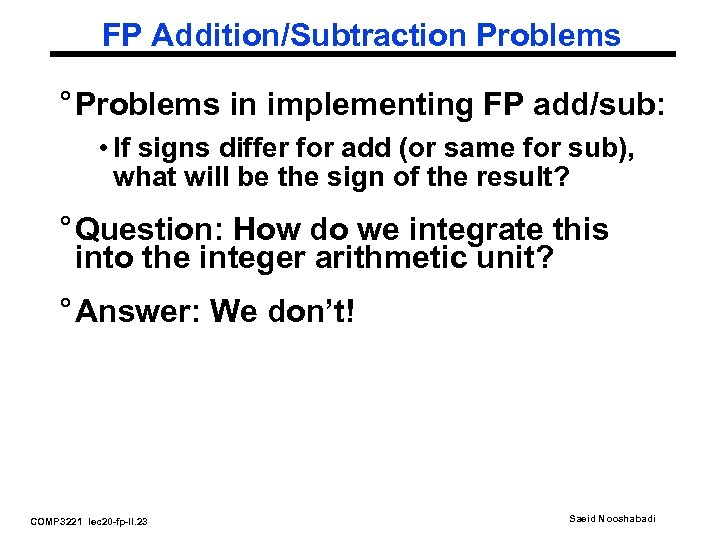FP Addition/Subtraction Problems ° Problems in implementing FP add/sub: • If signs differ for add (or same for sub), what will be the sign of the result? ° Question: How do we integrate this into the integer arithmetic unit? ° Answer: We don’t! COMP 3221 lec 20 -fp-II. 23 Saeid Nooshabadi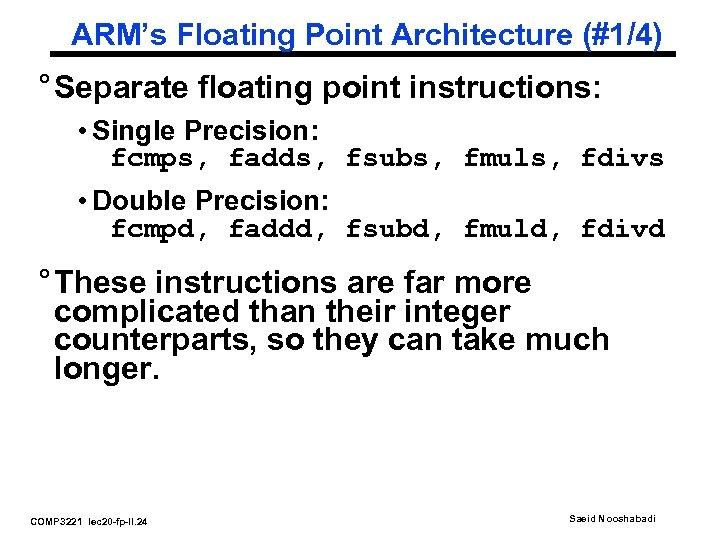ARM’s Floating Point Architecture (#1/4) ° Separate floating point instructions: • Single Precision: fcmps, fadds, fsubs, fmuls, fdivs • Double Precision: fcmpd, faddd, fsubd, fmuld, fdivd ° These instructions are far more complicated than their integer counterparts, so they can take much longer. COMP 3221 lec 20 -fp-II. 24 Saeid Nooshabadi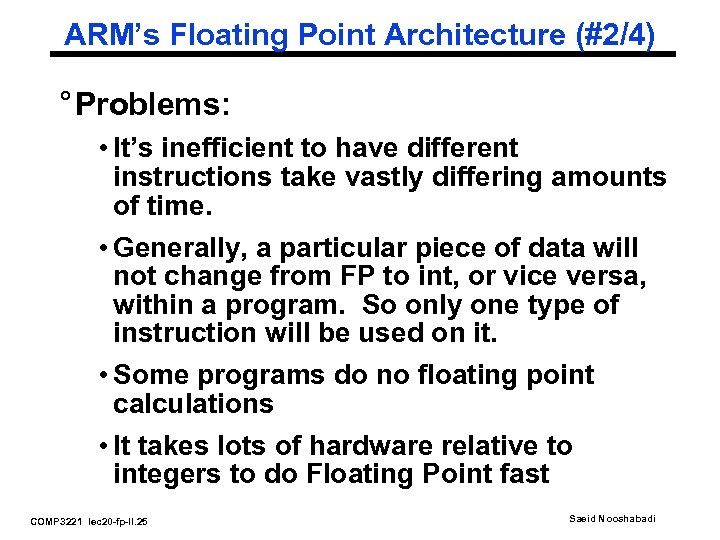ARM’s Floating Point Architecture (#2/4) ° Problems: • It’s inefficient to have different instructions take vastly differing amounts of time. • Generally, a particular piece of data will not change from FP to int, or vice versa, within a program. So only one type of instruction will be used on it. • Some programs do no floating point calculations • It takes lots of hardware relative to integers to do Floating Point fast COMP 3221 lec 20 -fp-II. 25 Saeid Nooshabadi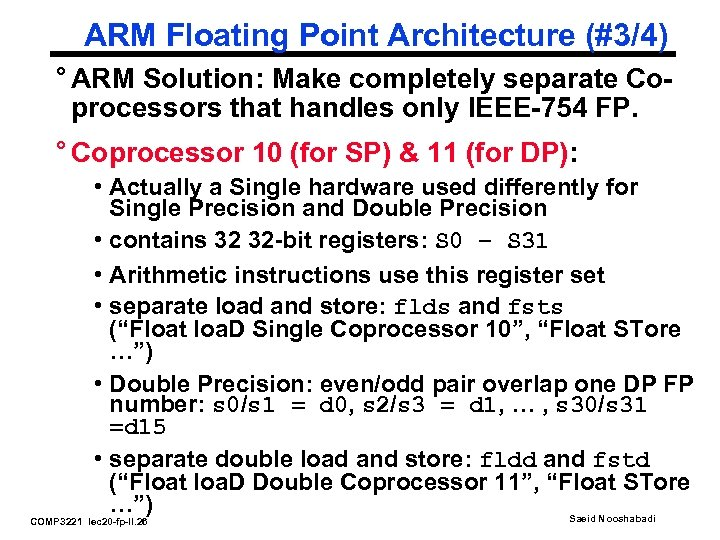ARM Floating Point Architecture (#3/4) ° ARM Solution: Make completely separate Coprocessors that handles only IEEE-754 FP. ° Coprocessor 10 (for SP) & 11 (for DP): • Actually a Single hardware used differently for Single Precision and Double Precision • contains 32 32 -bit registers: S 0 – S 31 • Arithmetic instructions use this register set • separate load and store: flds and fsts (“Float loa. D Single Coprocessor 10”, “Float STore …”) • Double Precision: even/odd pair overlap one DP FP number: s 0/s 1 = d 0, s 2/s 3 = d 1, … , s 30/s 31 =d 15 • separate double load and store: fldd and fstd (“Float loa. D Double Coprocessor 11”, “Float STore …”) Saeid Nooshabadi COMP 3221 lec 20 -fp-II. 26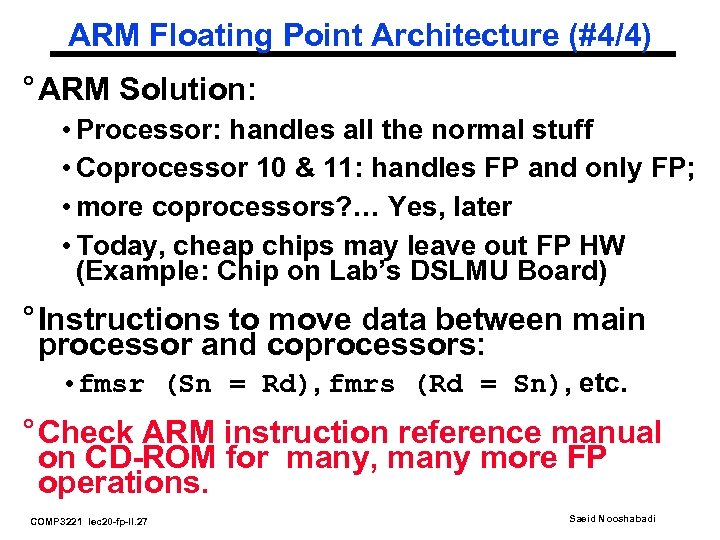ARM Floating Point Architecture (#4/4) ° ARM Solution: • Processor: handles all the normal stuff • Coprocessor 10 & 11: handles FP and only FP; • more coprocessors? … Yes, later • Today, cheap chips may leave out FP HW (Example: Chip on Lab’s DSLMU Board) ° Instructions to move data between main processor and coprocessors: • fmsr (Sn = Rd), fmrs (Rd = Sn), etc. ° Check ARM instruction reference manual on CD-ROM for many, many more FP operations. COMP 3221 lec 20 -fp-II. 27 Saeid Nooshabadi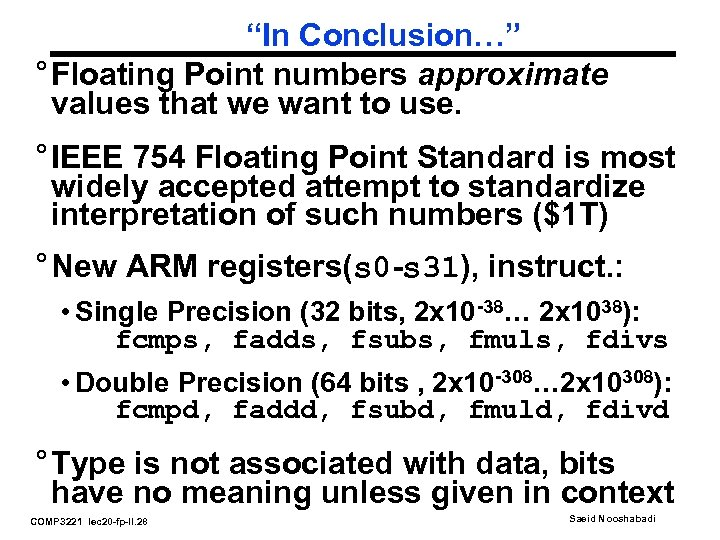“In Conclusion…” ° Floating Point numbers approximate values that we want to use. ° IEEE 754 Floating Point Standard is most widely accepted attempt to standardize interpretation of such numbers (\$1 T) ° New ARM registers(s 0 -s 31), instruct. : • Single Precision (32 bits, 2 x 10 -38… 2 x 1038): fcmps, fadds, fsubs, fmuls, fdivs • Double Precision (64 bits , 2 x 10 -308… 2 x 10308): fcmpd, faddd, fsubd, fmuld, fdivd ° Type is not associated with data, bits have no meaning unless given in context COMP 3221 lec 20 -fp-II. 28 Saeid Nooshabadi